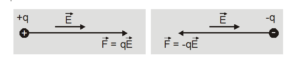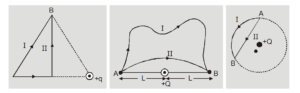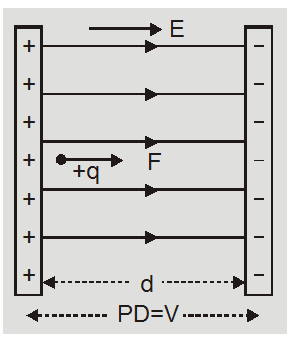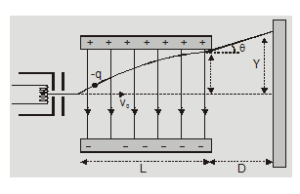Download the eSaral app and start learning from Kota's top IITians and doctors.

# Motion of charged particle in electric field - Class 12 - eSaral

Hey, do you want to learn about the motion of charged particle in electric field? If yes. Then keep reading

## Motion of a charged particle in an Electric Field

In case of motion of a charged particle in an electric field:
1. A point charge experiences a force, whether it is at rest or in motion $\overrightarrow{\mathrm{F}}=\mathrm{q} \overrightarrow{\mathrm{E}}$
2. The direction of force is parallel to the field if the charge is positive and opposite to the field if the charge is negative.3. The electric field is conservative so work is done is independent of path and work done in moving a point charge q between two fixed points having a potential difference V is equal to,$W_{A B}=-U_{A B}=q\left(V_{B}-V_{A}\right)=q V$
4. Work is done in moving a charged particle in an electric field unless the points are at the same potential5. When a charged particle is accelerated by a uniform or non-uniform electric field then by work-energy theorem

$\Delta K E=W$so $\frac{1}{2} m v^{2}-\frac{1}{2} m u^{2}=q V$

Or final velocity$v=\sqrt{\left[u^{2}+\frac{2 q V}{m}\right]}$

If the charged particle is initially at rest, then $v=\sqrt{\frac{2 q V}{m}}$

If the field is uniform $E=(\mathrm{V} / \mathrm{d})$ so $v=\sqrt{\frac{2 q E d}{m}}$

6. In motion of a charged particle in a uniform electric field if the force of gravity does not exist or is balanced by some other force say reaction or neglected then$\vec{a}=\frac{\vec{F}}{m}=\frac{\overrightarrow{q E}}{m}=$ constant
[as $\vec{F}=\overrightarrow{q E}]$Here equations of motion are valid.

• If the particle is initially at rest then from v = u + at, we get $v=a t=\frac{q E}{m} t$ And from Eqn.$s=u t+\frac{1}{2} a t^{2}$

we get $\quad s=\frac{1}{2} a t^{2}=\frac{1}{2} \frac{q E}{m} t^{2}$

The motion is accelerated translatory with $\mathrm{a} \propto \mathrm{t}^{\circ}$ ; $v \propto t$ and $s \propto t^{2}$Here $W=\Delta \mathrm{KE}=\frac{1}{2} \mathrm{mv}^{2}=\frac{1}{2} \mathrm{~m}\left[\frac{\mathrm{q} \mathrm{E}}{\mathrm{m}} \mathrm{t}\right]^{2}$

also

$W=q E d=q V$
• If the particle is projected perpendicular to the field with an initial velocity $\mathrm{V}_{0}$From Eqn. v = u + at

and

$s=u t+\frac{1}{2} a t^{2}$

$\mathrm{u}_{\mathrm{x}}=\mathrm{v}_{0}$

and $\quad a_{x}=0$

$\mathrm{u}_{\mathrm{x}}=\mathrm{v}_{0}=\mathrm{const.}$

and $\quad x=v_{0} t$

for motion along y-axis as $u_{y}=0$

and $a_{y}=\frac{q E}{m}$$v_{y}=\left[\frac{q E}{m}\right] t$

and $\quad y=\frac{1}{2}\left[\frac{q E}{m}\right] t^{2}$

So eliminating t between equation for x and y, we have

$\mathrm{y}=\frac{\mathrm{q} \mathrm{E}}{2 \mathrm{~m}}\left[\frac{\mathrm{x}}{\mathrm{v}_{0}}\right]^{2}=\frac{\mathrm{q} \mathrm{E}}{2 \mathrm{mv}_{0}^{2}} \mathrm{x}^{2}$

If the particle is projected perpendicular to the field the path is a parabola.

So, that's all from this article. I hope you get the idea about the motion of charged particles in electric field. If you liked this article then please share it with your friends. If you have any confusion related to this topic then feel free to ask in the comments section down below.

For a better understanding of this chapter, please check the detailed notes of the Electrostatics . To watch Free Learning Videos on physics by Saransh Gupta sir Install the eSaral App.